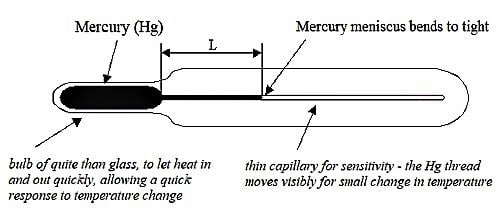# What do you mean by Sensitivity of a Thermometer?

The sensitivity of a thermometer refers to the minor temperature transform that can be detected or considered. The sensitivity of a thermometer is defined as the increase in the length of the mercury column per unit increase in temperature. The range of a thermometer, on the other hand, refers to the dissimilarity between the maximum and the minimum temperature. It is calculated in meter/degree Celsius.

For measuring temperature a sensitive thermometer is needed. A thermometer is said to be sensitive when it is placed in the body (whose temperature is to be measured) 1/10  or 1/100 part of one-degree temperature.Fig: Sensitivity of a Thermometer

Under the following conditions, a liquid in glass thermometer becomes sensitive-

• For a small change of temperature more change in the height of the liquid column.
• The bulb should be of moderate size and the capillary tube should not be extremely narrow. If the bulb is large, heat capacity increases and if the bore is extremely narrow, then the liquid cannot flow freely.

The sensitivity of a thermometer can be increased by –

• Using a thermometer with a smaller bulb – A smaller bulb contains less mercury and therefore absorbs heat in a shorter moment in time.
• A glass bulb with thinner wall – Heat can be transferred to the bulb straightforwardly.
• Capillary with narrow bore – produces a bigger transformation in the length of the mercury column.

Different Explanation –

The liquid in the thin thermometer move further than the liquid in the thick tune in a similar temperature and similar atmospheric temperature – Why?

Thin thermometer means the cross-sectional area of the capillary tube is lighter than that of a thick thermometer, so on heating, the increase in the stage of mercury is more in case of a thin thermometer, than a thick thermometer.

Suppose, on heating mercury by ‘x’ degree, its new size becomes ‘V’

Now, for a thin thermometer, V = A × l (where, A is the cross-sectional area and ‘l’ is the length, that indicates the increase of mercury in the tube)

And, for a thick thermometer, V = A’ × l’ (where A’ is the cross-sectional area and l’ is the rise in mercury stage)

Now, we can write,

l/l’ = A’/A

As, A’ > A

So, l > l’

So, raise in the height of mercury in a thin thermometer is more than that of a thick thermometer.

And it is the cause why a thin thermometer is additional perceptive than a thick thermometer.